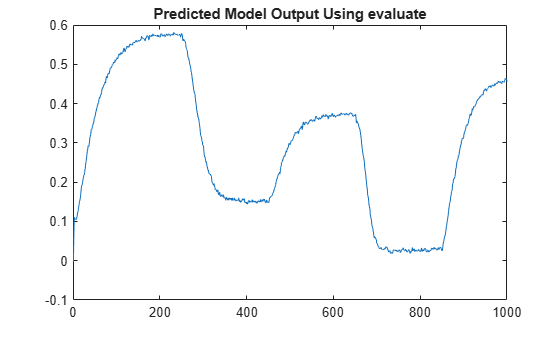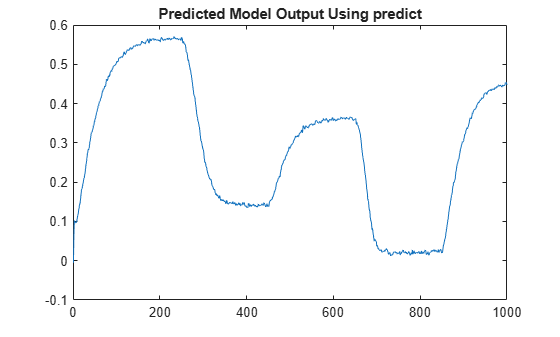getreg

Regressor expressions and numerical values in nonlinear ARX model

Syntax

Rs = getreg(model)
Rm = getreg(model,data)
Rm = getreg(model,data,init)
Rm = getreg(___,'Type',regressorType)

Description

Rs = getreg(model) returns expressions for computing regressors in the nonlinear ARX model. model is an idnlarx object. A typical use of the regression matrices built by getreg is to generate input data when you want to evaluate the output of a mapping function such as idWaveletNetwork using evaluate. For example, the following pair of commands evaluates the output of a mapping function model.

Regressor_Value = getreg(model,data,'z')
y = evaluate(model.OutputFcn,RegressorValue)
These commands are equivalent to the command:
y = predict(model,data,1,predictOptions('InitialCondition','z'))

Rm = getreg(model,data) returns regressor values as a timetable for the specified input/output data set data.

Rm = getreg(model,data,init) uses the initial conditions that are specified in init. The first N rows of each regressor matrix depend on the initial states init, where N is the maximum delay in the regressors (see getDelayInfo).

Rm = getreg(___,'Type',regressorType) returns the names of the regressors of the specified regressorType. For example, use the command Rm = getreg(model,'Type','input') to return the names of only the input regressors.

Input Arguments

data

iddata object containing measured data or numeric matrix that contains the values of the output and input variables in the order [model.OutputName model.InputName].

init

• 'z' (default) specifies zero initial state.

• NaN denotes unknown initial conditions.

• Real column vector containing the initial state values. For more information on initial states, see Definition of idnlarx States in idnlarx. For multiple-experiment data, this is a matrix where each column specifies the initial state of the model corresponding to that experiment.

• iddata object containing input and output samples at time instants before to the first sample in data. When the iddata object contains more samples than the maximum delay in the model, only the most recent samples are used. The number of samples required is equal to max(getDelayInfo(model)).

model

iddata object representing nonlinear ARX model.

regressorType

Type of regressor to return, specified as one of the following:

• 'all' (default) — All regressors

• 'input' — Only input regressors

• 'output' — Only output regressors

• 'standard' — Only linear and polynomial regressors

• 'custom' — Only custom regressors

Output Arguments

Rm

timetable of regressor values for all or a specified subset of regressors. Each column in Rm contains as many rows as there are data samples. For a model with nr regressors, Rm contains one column for each regressor. When data contains multiple experiments, Rm is a cell array where each element corresponds to a timetable of regressor values for an experiment.

Rs

Regressor expressions represented as a cell array of character vectors. For example, the expression 'u1(t-2)' computes the regressor by delaying the input signal u1 by two time samples. Similarly, the expression 'y2(t-1)' computes the regressor by delaying the output signal y2 by one time sample.

The order of regressors in Rs corresponds to regressor indices in the idnlarx object property model.RegressorUsage.

Examples

collapse all

Load sample data u and y.

Ts = 0.2;

Sample time is 0.2 sec.

Create data object and use first 1000 samples for estimation.

z = iddata(y,u,Ts);
ze = z(1:1000);

Estimate nonlinear ARX model.

model = nlarx(ze,[3 2 1]);

Get regressor expressions.

Rs = getreg(model)
Rs = 5x1 cell
{'y1(t-1)'}
{'y1(t-2)'}
{'y1(t-3)'}
{'u1(t-1)'}
{'u1(t-2)'}

Get regressor values.

Rm = getreg(model,ze)
Rm=1000×5 timetable
Time      y1(t-1)     y1(t-2)     y1(t-3)     u1(t-1)    u1(t-2)
_______    ________    ________    ________    _______    _______

0.2 sec           0           0           0       0          0
0.4 sec      0.1003           0           0      10          0
0.6 sec    0.094621      0.1003           0      10         10
0.8 sec    0.084424    0.094621      0.1003      10         10
1 sec      0.081449    0.084424    0.094621      10         10
1.2 sec     0.08546    0.081449    0.084424      10         10
1.4 sec    0.083002     0.08546    0.081449      10         10
1.6 sec     0.08443    0.083002     0.08546      10         10
1.8 sec    0.092793     0.08443    0.083002      10         10
2 sec      0.099804    0.092793     0.08443      10         10
2.2 sec     0.10559    0.099804    0.092793      10         10
2.4 sec      0.1081     0.10559    0.099804      10         10
2.6 sec     0.12108      0.1081     0.10559      10         10
2.8 sec     0.12404     0.12108      0.1081      10         10
3 sec       0.13551     0.12404     0.12108      10         10
3.2 sec     0.13405     0.13551     0.12404      10         10
⋮

Evaluate and plot model output for one-step-prediction.

Y = evaluate(model.OutputFcn,Rm.Variables);
plot(1:1000,Y)
title('Predicted Model Output Using evaluate')The previous result is equivalent to the result obtained by using predict in the following commands.

Y_p = predict(model,ze,1,'z');
Y = Y_p.OutputData;
plot(Y)
title('Predicted Model Output Using predict')Compatibility Considerations

expand all

Behavior changed in R2021a